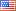## Recent progress in mathematical analysis of vortex sheets

Sijue Wu, University of Michigan, Ann ArborWe consider the motion of the interface separating two domains of the same fluid that moves with different velocities along the tangential direction of the interface. The evolution of the interface (the vortex sheet) is governed by the Birkhoff–Rott equations. We investigate the specific nature of the vortex sheet motion, in particular after the singularity formation; and consider the question of the weakest possible assumptions such that the Birkhoff–Rott equation makes sense. This leads us to introduce chord-arc curves to this problem. We present three results. The first can be stated as the following: Assume that the Birkhoff–Rott equation has a solution in a weak sense and that the vortex strength is bounded away from 0 and infinity. Moreover, assume that the solution gives rise to a vortex sheet curve that is chord-arc. Then the curve is automatically smooth, in fact analytic, for fixed time. The second and third results demonstrate that the Birkhoff–Rott equation can be solved if and only if ONLY half the initial data is given.

Transparencies in PDF: 3.5 MB.